# Phys 102 Lecture 2 Coulombs Law Electric Dipoles

• Slides: 23Phys 102 – Lecture 2 Coulomb’s Law & Electric Dipoles 1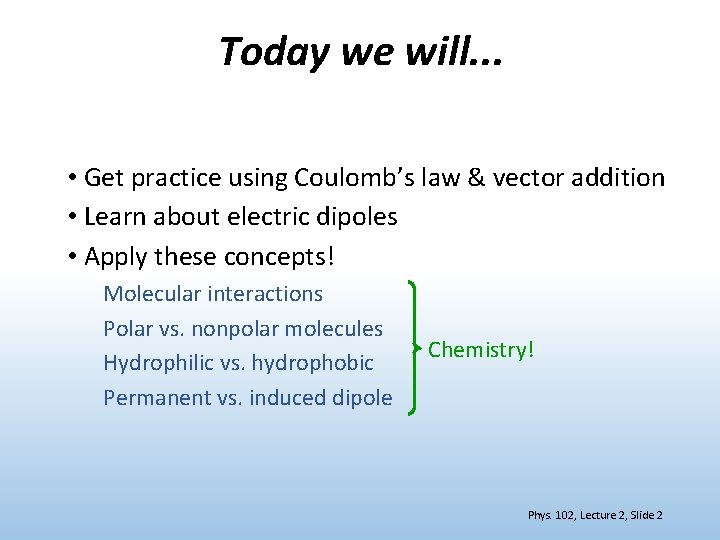Today we will. . . • Get practice using Coulomb’s law & vector addition • Learn about electric dipoles • Apply these concepts! Molecular interactions Polar vs. nonpolar molecules Hydrophilic vs. hydrophobic Permanent vs. induced dipole Chemistry! Phys. 102, Lecture 2, Slide 2Recall: Coulomb’s Law Force between charges q 1 and q 2 separated a distance r: “Coulomb constant” e ud it n ag M “Force on q 1 due to q 2” re Di io ct “Permittivity of free space” n Opposite charges attract, like charges repel Phys. 102, Lecture 2, Slide 3Superposition principle Total force on charge due to other charges = sum of individual forces Ex: what is the force on q 1 due to q 2, q 3, and q 4? q 1 q 2 q 3 q 4 Order does not matter! Phys. 102, Lecture 2, Slide 4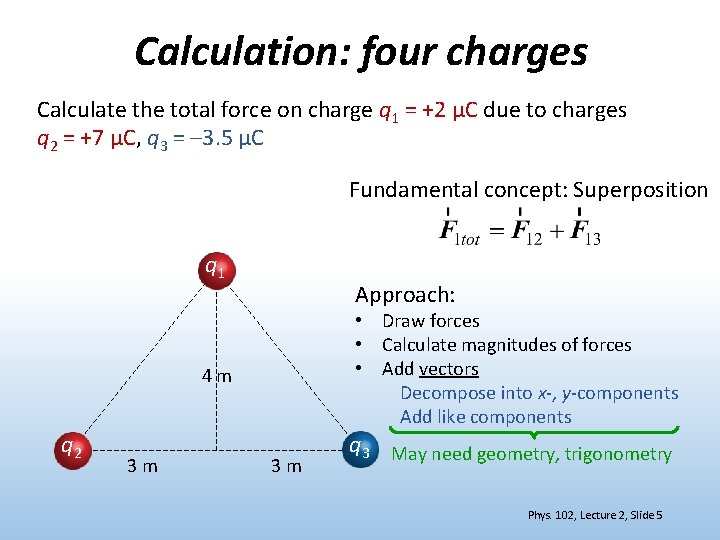Calculation: four charges Calculate the total force on charge q 1 = +2 μC due to charges q 2 = +7 μC, q 3 = – 3. 5 μC Fundamental concept: Superposition q 1 Approach: • Draw forces • Calculate magnitudes of forces • Add vectors Decompose into x-, y-components Add like components 4 m q 2 3 m 3 m q 3 May need geometry, trigonometry Phys. 102, Lecture 2, Slide 5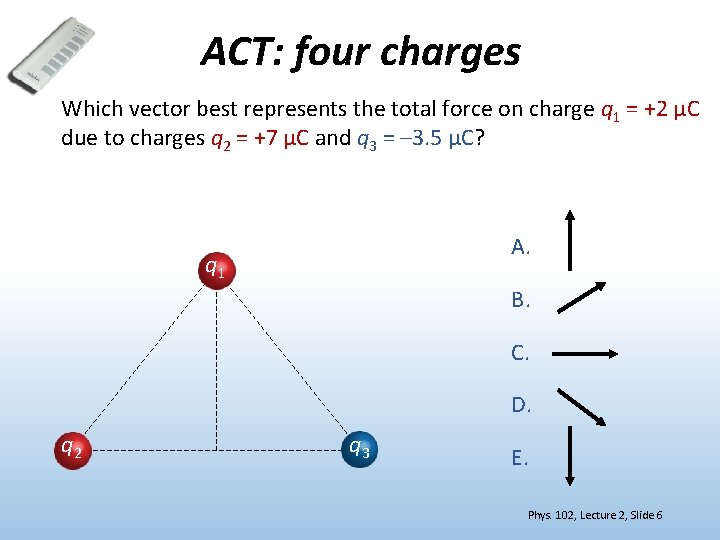ACT: four charges Which vector best represents the total force on charge q 1 = +2 μC due to charges q 2 = +7 μC and q 3 = – 3. 5 μC? A. q 1 B. C. D. q 2 q 3 E. Phys. 102, Lecture 2, Slide 6Calculation: four charges Calculate the total force on charge q 1 = +2 μC due to charges q 2 = +7 μC and q 3 = – 3. 5 μC • Calculate magnitudes of forces q 1 4 m q 2 3 m 3 m q 3 Phys. 102, Lecture 2, Slide 7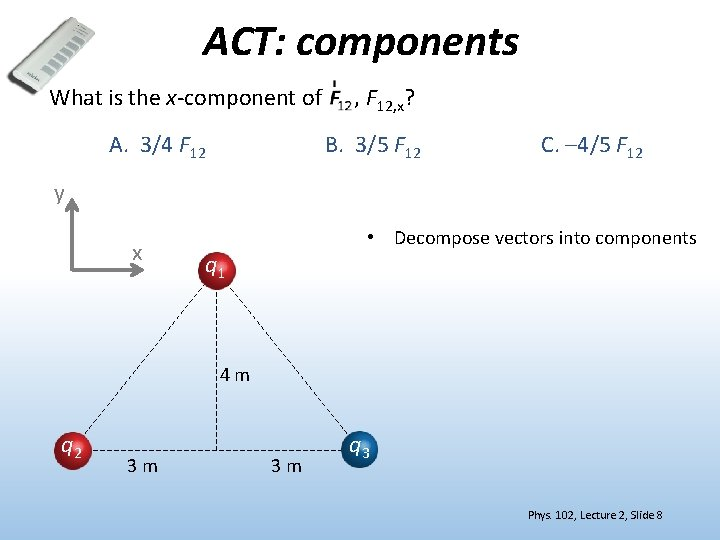ACT: components What is the x-component of A. 3/4 F 12 , F 12, x? B. 3/5 F 12 C. – 4/5 F 12 y x • Decompose vectors into components q 1 4 m q 2 3 m 3 m q 3 Phys. 102, Lecture 2, Slide 8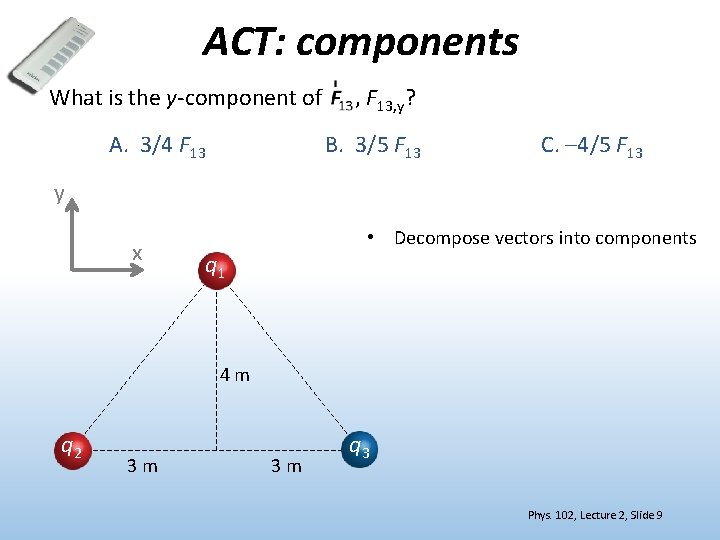ACT: components What is the y-component of A. 3/4 F 13 , F 13, y? B. 3/5 F 13 C. – 4/5 F 13 y x • Decompose vectors into components q 1 4 m q 2 3 m 3 m q 3 Phys. 102, Lecture 2, Slide 9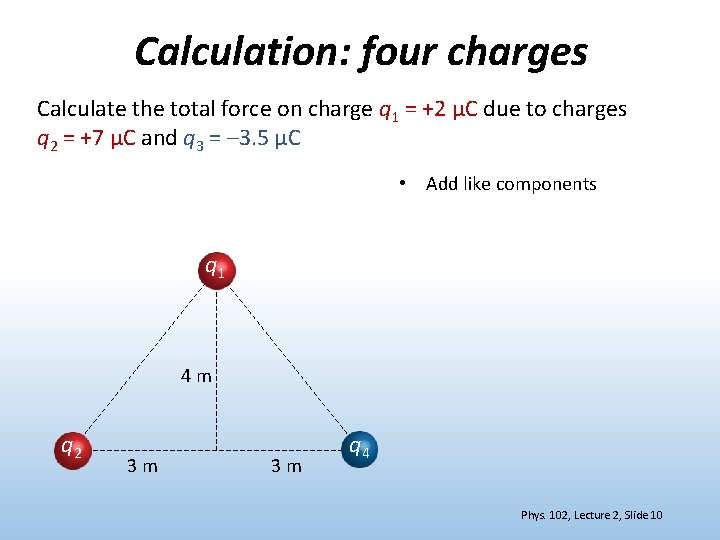Calculation: four charges Calculate the total force on charge q 1 = +2 μC due to charges q 2 = +7 μC and q 3 = – 3. 5 μC • Add like components q 1 4 m q 2 3 m 3 m q 4 Phys. 102, Lecture 2, Slide 10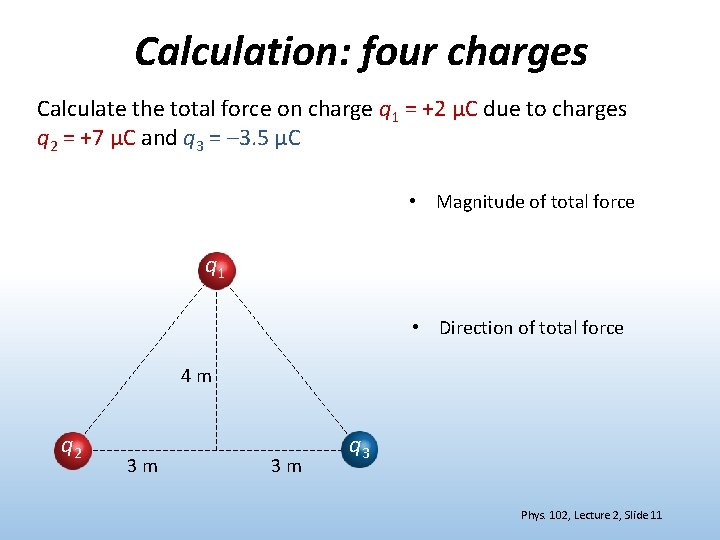Calculation: four charges Calculate the total force on charge q 1 = +2 μC due to charges q 2 = +7 μC and q 3 = – 3. 5 μC • Magnitude of total force q 1 • Direction of total force 4 m q 2 3 m 3 m q 3 Phys. 102, Lecture 2, Slide 11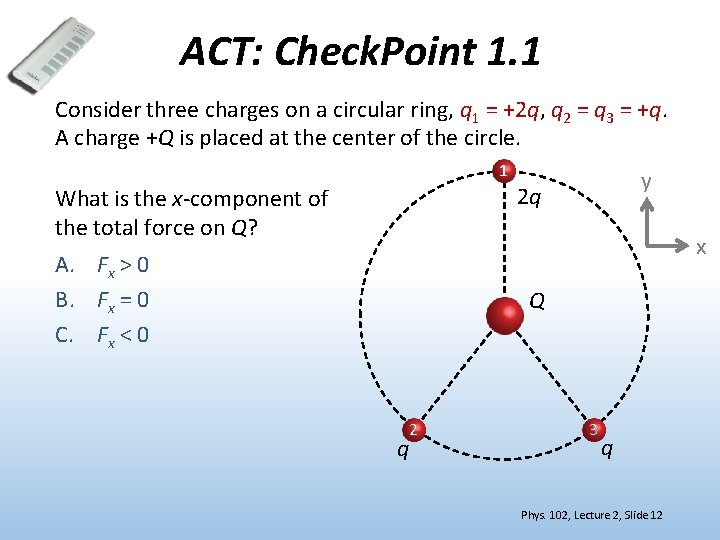ACT: Check. Point 1. 1 Consider three charges on a circular ring, q 1 = +2 q, q 2 = q 3 = +q. A charge +Q is placed at the center of the circle. 1 What is the x-component of the total force on Q? y 2 q x A. Fx > 0 B. Fx = 0 C. Fx < 0 Q 2 q 3 q Phys. 102, Lecture 2, Slide 12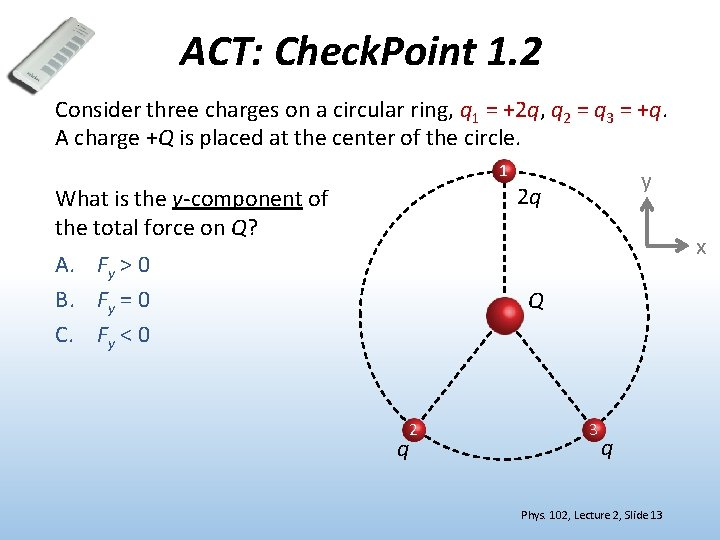ACT: Check. Point 1. 2 Consider three charges on a circular ring, q 1 = +2 q, q 2 = q 3 = +q. A charge +Q is placed at the center of the circle. 1 What is the y-component of the total force on Q? y 2 q x A. Fy > 0 B. Fy = 0 C. Fy < 0 Q 2 q 3 q Phys. 102, Lecture 2, Slide 13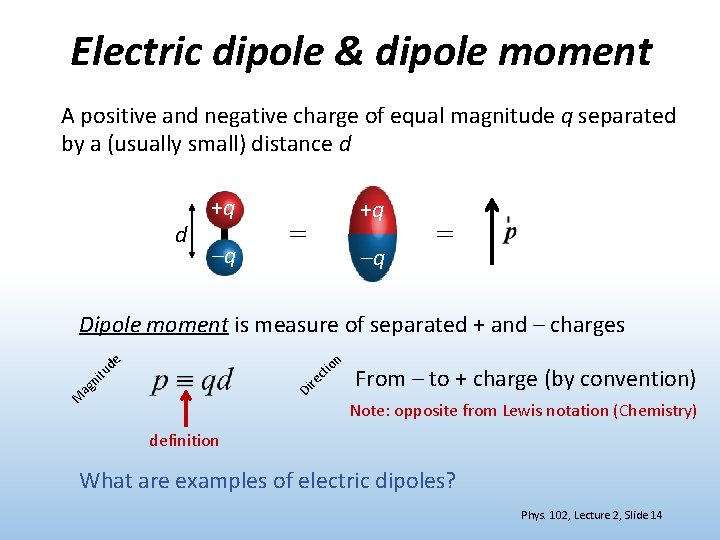Electric dipole & dipole moment A positive and negative charge of equal magnitude q separated by a (usually small) distance d d +q –q +q = –q = Dipole moment is measure of separated + and – charges n ag de n io t ec itu r Di M From – to + charge (by convention) Note: opposite from Lewis notation (Chemistry) definition What are examples of electric dipoles? Phys. 102, Lecture 2, Slide 14Molecular dipole Electrons are not shared equally between chemically bonded atoms Charge imbalance creates a bond dipole Ex: HF (hydrofluoric acid) Ex: H 20 (water) Slightly negative Slightly positive δ+ 2δ- δδ+ δ+ Polar ptot > 0 Ex: CO 2 (carbon dioxide) 2δ+ δ- δ- Nonpolar ptot = 0 Phys. 102, Lecture 2, Slide 15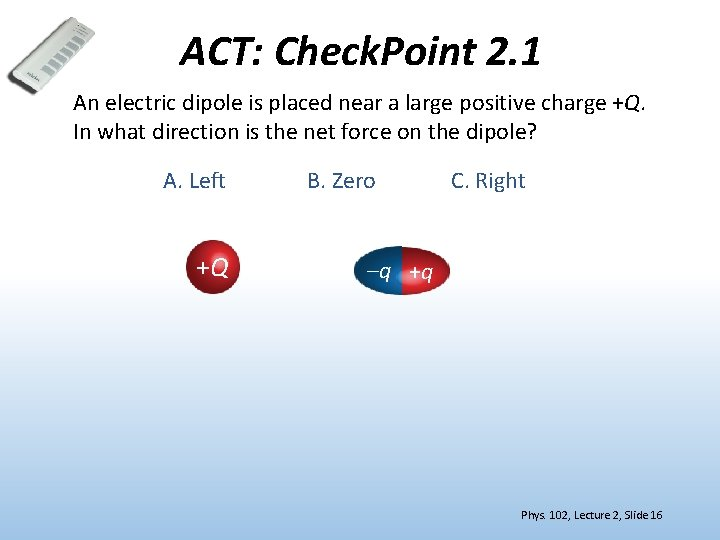ACT: Check. Point 2. 1 An electric dipole is placed near a large positive charge +Q. In what direction is the net force on the dipole? A. Left +Q B. Zero C. Right –q +q Phys. 102, Lecture 2, Slide 16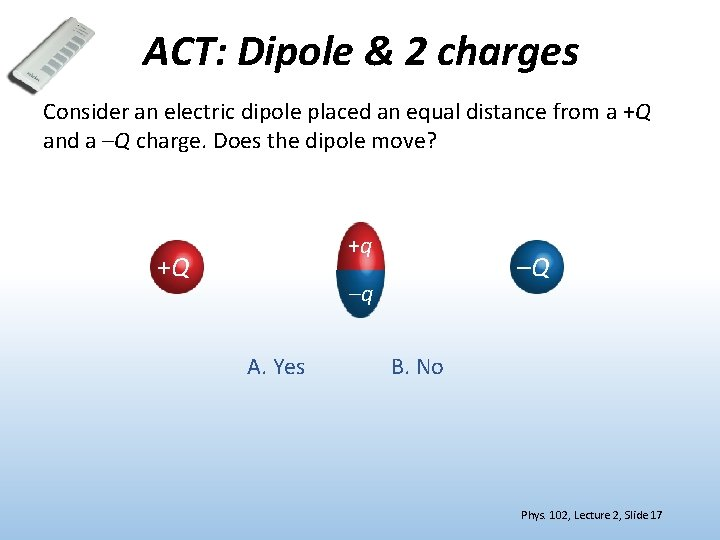ACT: Dipole & 2 charges Consider an electric dipole placed an equal distance from a +Q and a –Q charge. Does the dipole move? +q +Q –Q –q A. Yes B. No Phys. 102, Lecture 2, Slide 17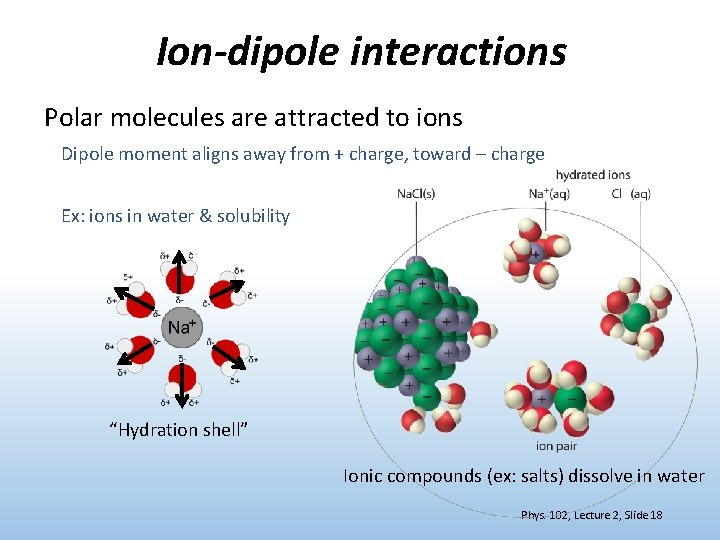Ion-dipole interactions Polar molecules are attracted to ions Dipole moment aligns away from + charge, toward – charge Ex: ions in water & solubility “Hydration shell” Ionic compounds (ex: salts) dissolve in water Phys. 102, Lecture 2, Slide 18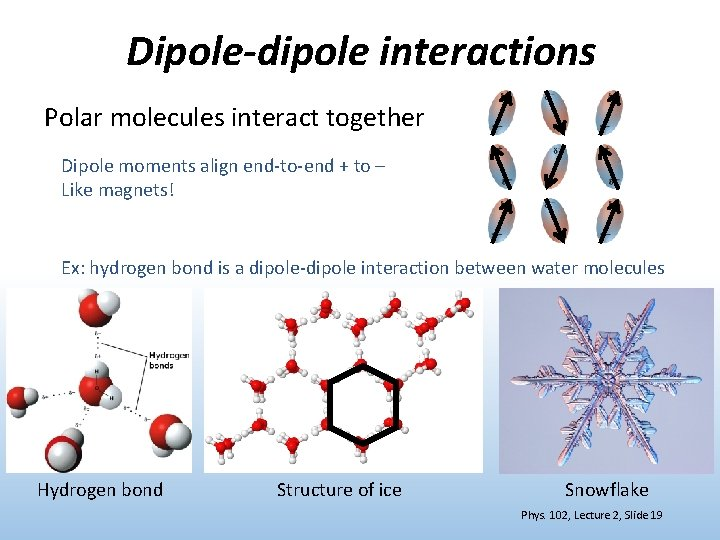Dipole-dipole interactions Polar molecules interact together Dipole moments align end-to-end + to – Like magnets! Ex: hydrogen bond is a dipole-dipole interaction between water molecules Hydrogen bond Structure of ice Snowflake Phys. 102, Lecture 2, Slide 19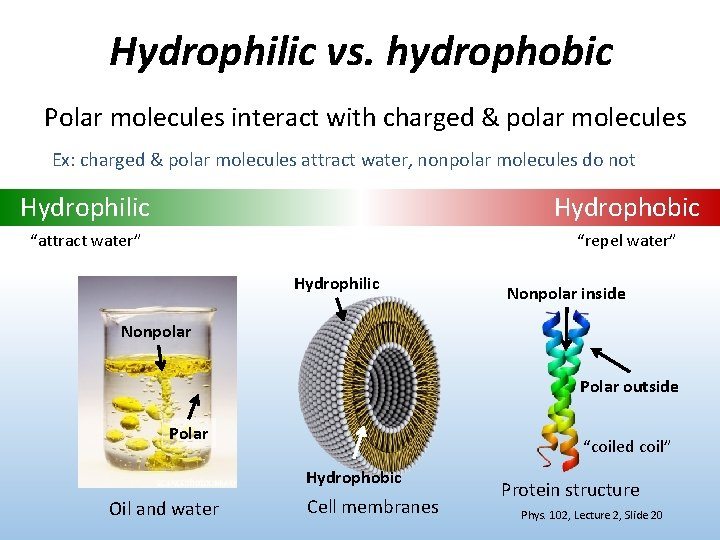Hydrophilic vs. hydrophobic Polar molecules interact with charged & polar molecules Ex: charged & polar molecules attract water, nonpolar molecules do not Hydrophilic Hydrophobic “attract water” “repel water” Hydrophilic Nonpolar inside Nonpolar Polar outside Polar “coiled coil” Hydrophobic Oil and water Cell membranes Protein structure Phys. 102, Lecture 2, Slide 20ACT: Charge & conductor An uncharged conducting sphere is placed next to a fixed + charge. What happens when the uncharged sphere is released? + DEMO A. Nothing B. Attracted to + sphere C. Repelled from + sphere Phys. 102, Lecture 2, Slide 21Molecular interactions Interactions between molecules are understood in terms of charges and electric dipoles interacting by Coulomb’s law Ion-dipole Dipole-induced dipole Induced dipoleinduced dipole? Ion-induced dipole Yes! Two nonpolar molecules can induce dipoles in each other and interact! London dispersion or van der Waals force Phys. 102, Lecture 2, Slide 22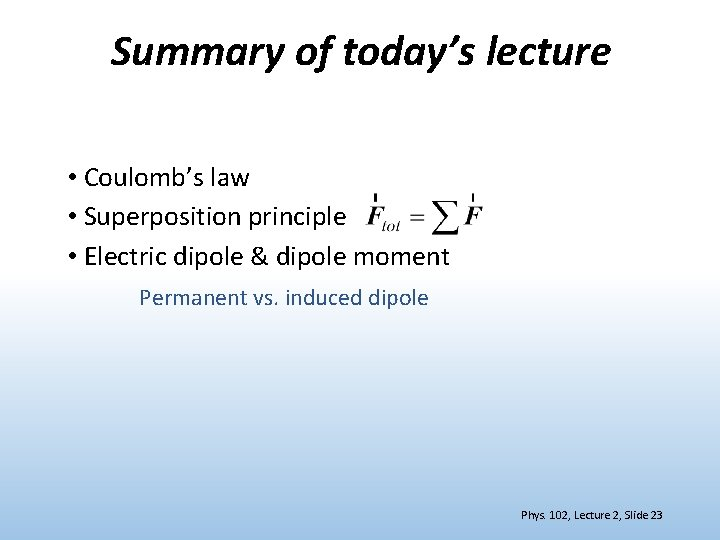Summary of today’s lecture • Coulomb’s law • Superposition principle • Electric dipole & dipole moment Permanent vs. induced dipole Phys. 102, Lecture 2, Slide 23